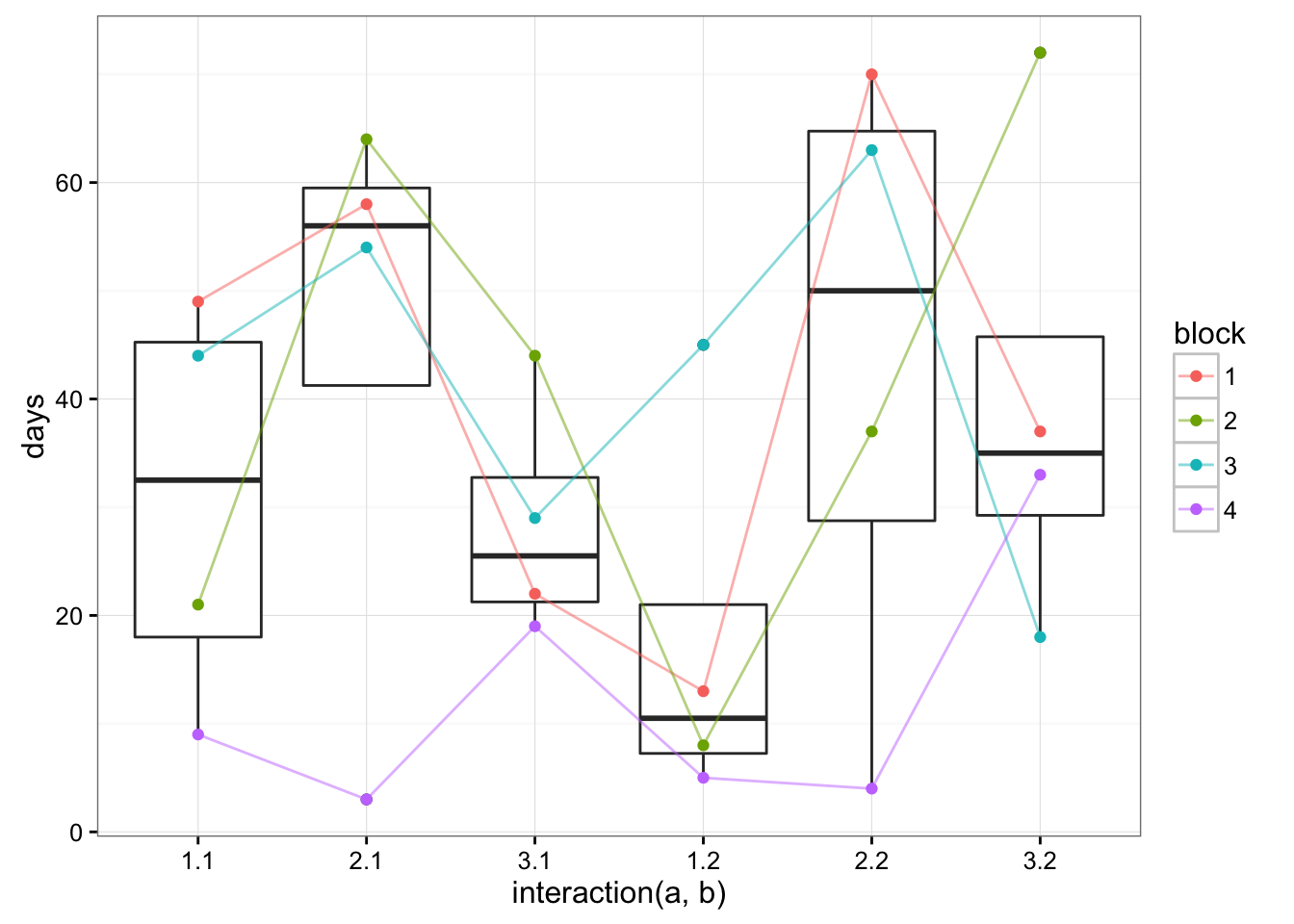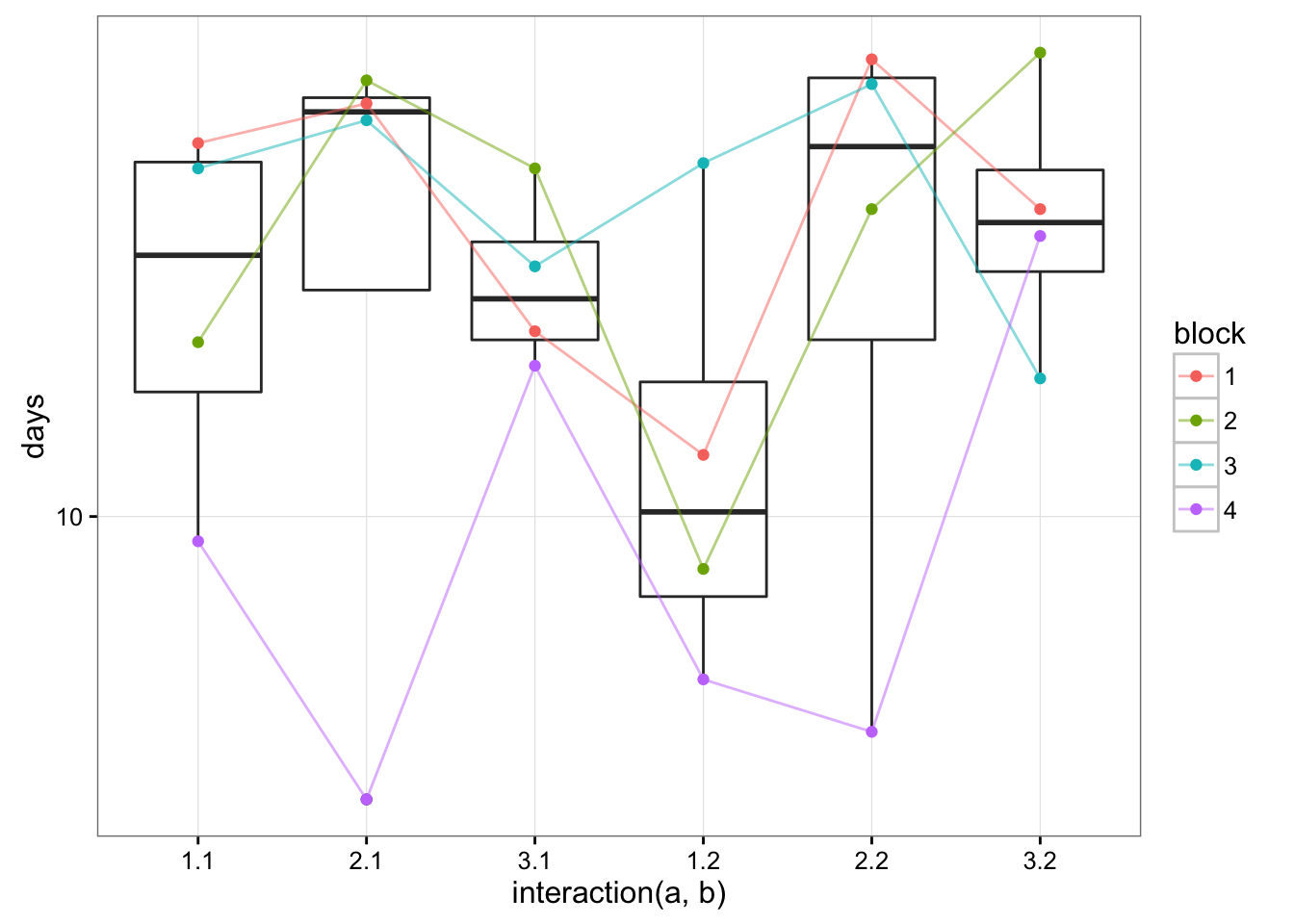This is a quick example of analyzing a small, fake data set on flowering times. The example comes from a presentation by Walt Stroup; there is also a description of the data set in Stroup’s book (Google Books excerpt).

The data for this example appear as Data Set 12.1 in the SAS Data and Program Library. The design uses four complete blocks with three units each. Treatment factor A has three levels, which are randomly assigned to units within blocks so that each level of A appears once per block. Each unit consists of two subunits. Treatment factor B has two levels. They are randomly assigned to subunits so that each level of B appears once in each unit within a block. Thus, the treatment is a 3x2 factorial and the study design is split-plot. The response variable, denoted DAYS in Data Set 12.1, is short for the number of days until the event of interest happens. These are simulated data …

(The SAS manual has data on a different (real) flowering-time data set from Cockerham & Weir …)

``````library("lme4")
library("nlme")
library("glmmTMB")
library("broom")
library("dotwhisker")
library("lsmeans")
library("ggplot2"); theme_set(theme_bw())
library("plyr") ## for ldply()
library("dplyr")
options(digits=5)``````

(Note that this requires cutting-edge/my forked Github versions of packages such as `broom`, `glmmTMB` …)

``````d2 <- data.frame(setNames(expand.grid(1:2,1:3,1:4)[3:1],c("block","a","b")),
days=as.integer(
c(49,13,58,70,22,37,21,8,64,37,44,72,44,45,54,63,29,18,
9,5,3,4,19,33)))
d2[,1:3] <- lapply(d2[,1:3],factor)``````
``````(gg1 <- ggplot(d2,aes(x=interaction(a,b),y=days))+geom_boxplot()+
geom_point(aes(colour=block))+
geom_line(aes(colour=block,group=block),alpha=0.5))``````Block #4 looks a little crappy … but otherwise there’s not too much going on here.

Things look a little better on a log scale, although not horribly so …

``gg1 + scale_y_log10()````````lmm_lme <- lme(days~a*b,random=~1|block/a,d2)
lmm_lmer <- lmer(days~a*b+(1|block/a),d2)``````

If we look at the `lsmeans` of this example, the estimates all match Stroup’s results exactly (they should, since these are just the group means!); the standard errors match as well. Stroup reports 9 degrees of freedom; `lme` reports `df=3` (an underestimate (??) based on the Pinheiro & Bates approximate algorithm??); `lmer` reports `df=10.61` (`lsmeans` calls `pbkrtest`’s Kenward-Roger approximation by default). This leads to large differences in the confidence intervals:

``````## lower CIs for `a=1; b=2`
17.75-11.2412*qt(0.975,c(lme=3,stroup=9,lmer=10.61))``````
``````##      lme   stroup     lmer
## -18.0245  -7.6794  -7.1031``````

## Gamma models

``````## use a:b-1 to get lsmeans==parameters
gamma_glmer <- glmer(days~a:b-1+(1|block/a),
data=d2,
``````comb <- rbind.fill(stroup_res,ldply(list(glmer=gamma_glmer,TMB=gamma_glmmTMB),